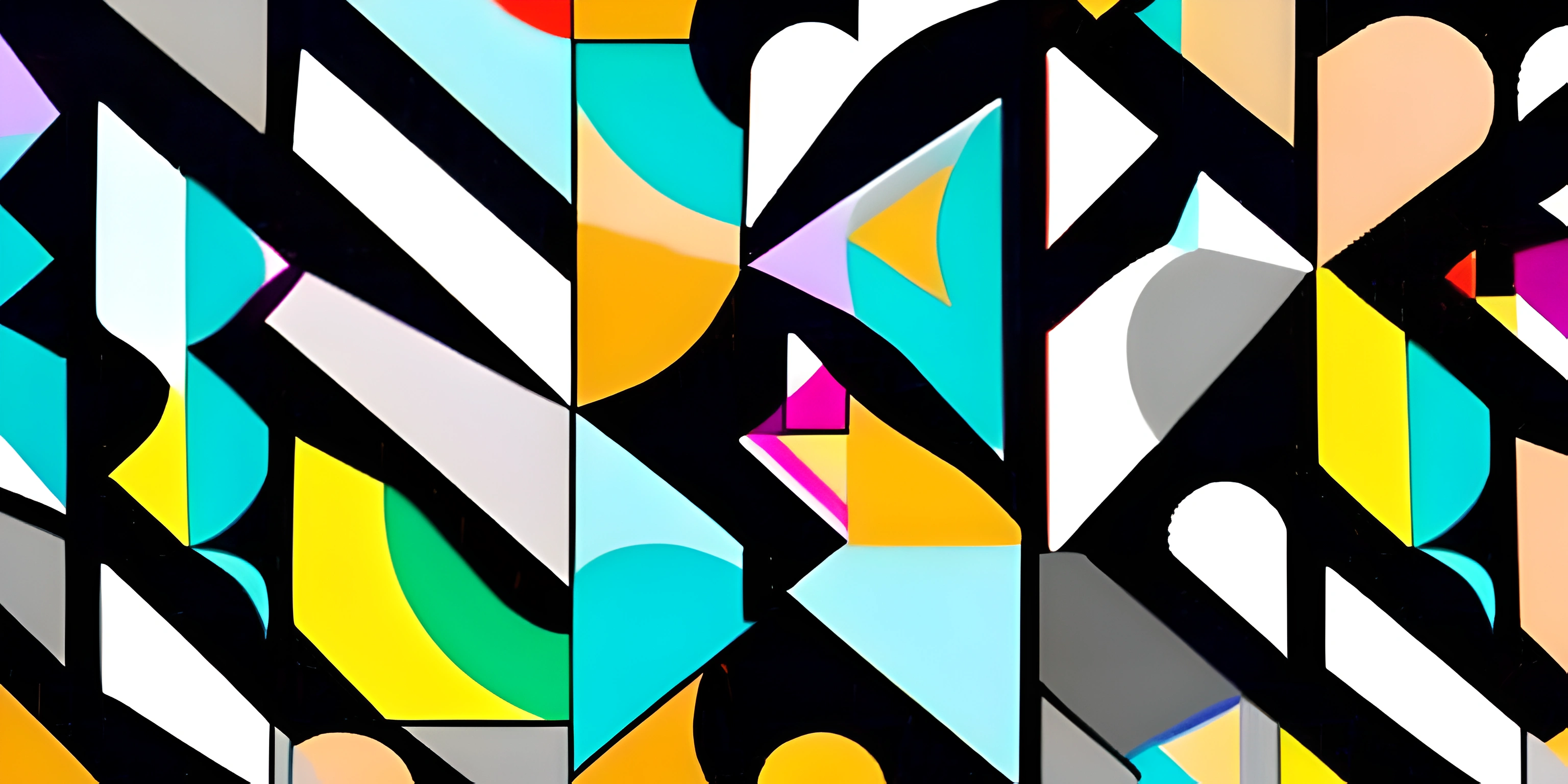# p5.js Animation BasicsNote: this page has been created with the use of AI. Please take caution, and note that the content of this page does not necessarily reflect the opinion of Cratecode.

Creating animations with p5.js is like becoming a digital magician. With a few lines of code, you can bring life to virtual shapes and colors. Before you know it, you'll have your own little world of moving pixels right at your fingertips.

## Setting up the Canvas

First things first, we need a stage for our animation. In p5.js, the stage is called the canvas. Let's create one using the `createCanvas()` function inside the `setup()` function:

``````function setup() {
createCanvas(800, 600);
}``````

This will create an 800x600 pixel canvas for our animation. Now that we have our stage, let's add some actors!

## Drawing Shapes

p5.js has a variety of functions for drawing shapes. We'll start simple with a circle. To draw a circle, use the `circle()` function, which takes three arguments: the x-coordinate, the y-coordinate, and the diameter.

``````function draw() {
circle(400, 300, 50);
}``````

This code will draw a circle with a diameter of 50 pixels in the center of the canvas. But it's not moving – let's make it dance!

## Animating Shapes

To animate our circle, we need to change its position over time. We'll use the `frameCount` variable provided by p5.js to make our circle move horizontally.

``````function draw() {
// Calculate the x-coordinate
let x = 400 + 100 * sin(frameCount * 0.05);

// Draw the circle
circle(x, 300, 50);
}``````

Our circle is now oscillating left and right. But wait, there's more! Let's make it bounce up and down as well.

``````function draw() {
// Calculate the x and y coordinates
let x = 400 + 100 * sin(frameCount * 0.05);
let y = 300 + 100 * cos(frameCount * 0.03);

// Draw the circle
circle(x, y, 50);
}``````

Our circle is now happily bouncing around like it's at a rave. But why stop at one circle? The party's just getting started!

## Creating Multiple Shapes

Let's add more circles to our animation. We can do this by using a `for` loop to draw multiple circles with different positions and sizes.

``````function draw() {
// Clear the background
background(255);

// Draw multiple circles
for (let i = 0; i < 10; i++) {
// Calculate the x and y coordinates
let x = 400 + 100 * sin(frameCount * 0.05 + i * 0.2);
let y = 300 + 100 * cos(frameCount * 0.03 + i * 0.2);

// Draw the circle
circle(x, y, 50 - i * 3);
}
}``````

We now have an army of circles dancing to the beat of our code. You're now a p5.js animation wizard!

## Final Thoughts

p5.js offers endless possibilities for creating animations. Experiment with different shapes, colors, and movements to make your animations truly unique. You're just a few lines of code away from creating your own mesmerizing digital art. So go forth and animate the digital world!

## FAQ

### What is p5.js and why is it useful for creating animations?

p5.js is a JavaScript library that makes it easy to create graphics and animations in a browser. It simplifies tasks like drawing shapes, working with colors, and handling user input. With its beginner-friendly syntax and extensive documentation, p5.js is perfect for anyone looking to add engaging visuals to their web-based projects.

### How can I set up a basic p5.js sketch for an animation?

To set up a basic p5.js sketch for an animation, you'll need to define two essential functions: `setup()` and `draw()`. The `setup()` function runs once at the beginning of your sketch, while `draw()` is continually called to create the animation. Here's a simple example to get you started:

``````function setup() {
createCanvas(600, 400);
}
function draw() {
background(255);
// Your animation code goes here
}``````

### How can I create a moving object in p5.js?

To create a moving object in p5.js, you'll need to update its position on the canvas in every frame of the animation. You can do this by using variables to store the object's position and adjusting their values inside the `draw()` function. Here's an example of a moving circle:

``````let x = 0;
function setup() {
createCanvas(600, 400);
}
function draw() {
background(255);
fill(0);
ellipse(x, height / 2, 50, 50);
x += 1;
}``````

### How can I control the speed of an animation in p5.js?

You can control the speed of an animation in p5.js by manipulating the frame rate using the `frameRate()` function. This function sets the number of times the `draw()` function is called per second. For example, calling `frameRate(30)` in the `setup()` function will set the frame rate to 30 frames per second (FPS).

``````function setup() {
createCanvas(600, 400);
frameRate(30);
}
function draw() {
// Your animation code goes here
}``````

### How can I make an object bounce off the edges of the canvas in p5.js?

To make an object bounce off the edges of the canvas, you'll need to reverse its direction when it reaches the edge. You can do this by using conditional statements and changing the sign of the object's speed. Here's an example of a bouncing circle:

``````let x = 0;
let speed = 2;
function setup() {
createCanvas(600, 400);
}
function draw() {
background(255);
fill(0);
ellipse(x, height / 2, 50, 50);
x += speed;
if (x < 0 || x > width) {
speed = -speed;
}
}``````